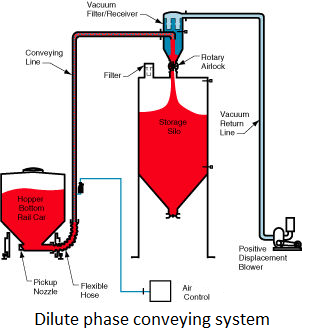Dilute phase conveying system calculation : Dilute phase conveying is a pneumatic conveying system in which the material is suspended in the air and transported through a pipeline. The system is widely used in industries such as cement, chemical, and food processing.Here are the basic steps to calculate the dilute phase conveying system:

1. Determine the material properties:

The properties of the material to be conveyed are essential to calculate the system. The bulk density, particle size, shape, and flow characteristics are some of the critical parameters to be considered.

1. Calculate the conveying parameters:

The following parameters need to be calculated to design the dilute phase conveying system:

• Air flow rate: The air flow rate is calculated by considering the material’s conveying velocity and the pressure drop in the pipeline. The minimum conveying velocity for a dilute phase system is around 15 m/s.
• Pipeline diameter: The pipeline diameter is calculated based on the material’s bulk density, conveying velocity, and air flow rate. The recommended ratio of the pipeline diameter to the material particle size is 20:1.
• Pressure drop: The pressure drop in the pipeline is a critical parameter to consider, as it affects the material’s conveying velocity and the system’s efficiency.
• Air velocity: The air velocity in the pipeline should be high enough to keep the material in suspension and low enough to avoid material degradation or pipeline wear.
1. Select the appropriate equipment:

The equipment needed for the dilute phase conveying system includes an air compressor, a pipeline, a material feeder, and an air-material separator. The selection of equipment depends on the material properties, the system’s capacity, and the available space.

1. Design the pipeline layout:

The pipeline layout should be designed based on the available space, the material’s flow characteristics, and the system’s capacity. The pipeline should be supported adequately and have enough bends and elbows to avoid material blockage.

Dilute phase conveying is a type of pneumatic conveying system used to transfer materials from one point to another using air as a conveying medium. The system operates by suspending particles in a high velocity air stream and transporting them through pipelines to the desired destination. Here’s a general overview of how to calculate a dilute phase conveying system:

1. Determine the Material Properties The first step in calculating a dilute phase conveying system is to determine the material properties. This includes the particle size, density, shape, and other properties that can affect how the material will behave in the system.
2. Calculate the Air Requirements The next step is to calculate the air requirements for the system. This involves determining the air velocity required to suspend the particles in the air stream and the amount of air flow needed to move the particles through the pipeline. The air velocity is typically between 15-30 m/s, and the air flow rate is calculated using the following equation:

Q = (G x L) / (ρ x V)

where: Q = air flow rate (m3/s) G = mass flow rate of material (kg/s) L = length of pipeline (m) ρ = density of air (kg/m3) V = velocity of air (m/s)

1. Determine the Pipeline Diameter The pipeline diameter is calculated based on the air flow rate and the required air velocity. The diameter is typically between 75-150 mm and is calculated using the following equation:

D = 4 x (Q / (π x V))

where: D = pipeline diameter (m) Q = air flow rate (m3/s) V = air velocity (m/s)

1. Calculate the Pressure Drop The pressure drop is the loss of pressure that occurs as the material and air move through the pipeline. This is calculated using the following equation:

ΔP = (f x L/D) x (G2/2) x ρ

where: ΔP = pressure drop (Pa) f = friction factor (determined based on the Reynolds number) L = length of pipeline (m) D = pipeline diameter (m) G = mass flow rate of material (kg/s) ρ = density of air (kg/m3)

1. Select the Air Compressor Based on the air flow rate and pressure drop, you can select the appropriate air compressor to power the system.

Note that the calculations and design considerations for a dilute phase conveying system can vary depending on the specific application and materials being transported. It’s important to consult with a qualified engineer or supplier to ensure the system is designed and installed correctly.

In conclusion, the dilute phase conveying system calculation requires a thorough understanding of the material properties and conveying parameters. The system design should consider the equipment selection and the pipeline layout to ensure the efficient and reliable operation of the system.

Translate »